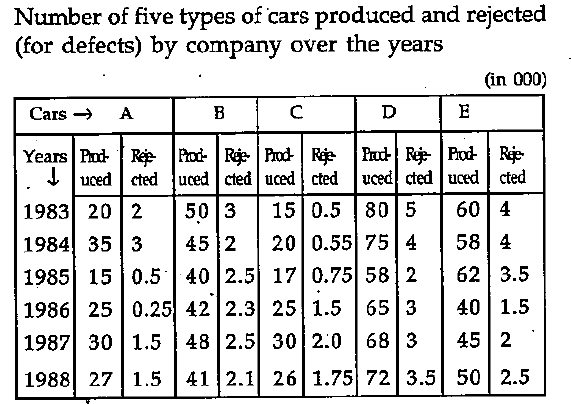# Numerical Reasoning - Numerical Reasoning Test 3

Instructions:

Study the following table carefully and answer the questions given below1:

In the case of B type car, which year was the ratio of rejection to production the highest among the given years?

 A. 1983 B. 1984 C. 1985 D. 1987 Answer Report Discuss Option: C Explanation : For B type car, the ratio of rejection to production in  1983 = 3/50 =0.060 1984 = 2/45 = 0.044 1985 = 2.5/40 = 0.063 1986 = 2.3/42 = 0.055 1987 = 2.5/48 = 0.052 1988 = 2.1/41 = 0.051 Click on Discuss to view users comments. Write your comments here:
2:

In the case of E type car, in which year was the ratio of rejection to production  the lowest among the given years?

 A. 1984 B. 1985 C. 1986 D. 1987 Answer Report Discuss Option: C Explanation : For E type car, the ratio of rejection to production in 1983 = 0.067 Similar figure for other ahead years are 0.069; 0.056, 0.038, 0.044, 0.050 respectively Click on Discuss to view users comments. Write your comments here:
3:

What was the difference in number of C type cars produced between 1984 and 1985?

 A. 200 B. 250 C. 3000 D. 2500 Answer Report Discuss Option: C Explanation : 20000-17000 = 3000 Click on Discuss to view users comments. Write your comments here:
4:

The acceptable E type cars in 1985 was what percent to that of 1984?

 A. 8 B. 106 C. 110 D. None of these Answer Report Discuss Option: D Explanation : (62-3.5) = k% of (58-4) k = (100*58.5)/54 = 108 Click on Discuss to view users comments. Write your comments here:
5:

In the year 1984, in the case of which cars was the rejection below five percent?

 A. A B. B or E C. B and C D. None of these Answer Report Discuss Option: C Explanation : Rejection percent in 1984 for type A = 300/35 = 8.57 and for other type cars the rejection percent is respectively 4.44 for type B; 2.75 for type C; 5.33 for type D; and 6.90 for type E Click on Discuss to view users comments. Write your comments here:

## Suggest an improvement

1. Take a free Numerical test before your next interview.
2. Take a numeracy psychometric test designed especially for CES and USB
3. Here you can practice questions for ceb numerical reasoning test.
4. These questions can be used for investment banking numerical test .
5. Here you will find questions answers for numeracy psychometric test.
6. This section provides you free online psychometric test.
7. These questions can be used for numerical assessment